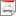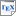[KM02] M. Kwiatkowska and R. Mehmood. Out-of-Core Solution of Large Linear Systems of Equations arising from Stochastic Modelling. In Proc. PAPM/PROBMIV'02, volume 2399 of LNCS, pages 135-151, Springer-Verlag. July 2002. [ps.gz] [bib] Downloads:ps.gz (126 KB)bib Notes: The original publication is available at link.springer.com. Links: [Google] [Google Scholar] [CiteSeer] Abstract. Many physical or computer systems can be modelled as Markov chains. A range of solution techniques exist to address the state-space explosion problem, encountered while analysing such Markov models. However, numerical solution of these Markov chains is impeded by the need to store the probability vector(s) explicitly in the main memory. In this paper, we extend the earlier out-of-core methods for the numerical solution of large Markov chains and introduce an algorithm which uses a disk to hold the probability vector as well as the matrix. We give experimental results of the implementation of the algorithm for a Kanban manufacturing system and a flexible manufacturing system. Next, we describe how the algorithm can be modified to exploit sparsity structure of a model, leading to better performance. We discuss two models, a cyclic server polling system and a workstation cluster system, in this context and present results for the polling models. We also introduce a new sparse matrix storage format which can provide 30% or more saving over conventional schemes.